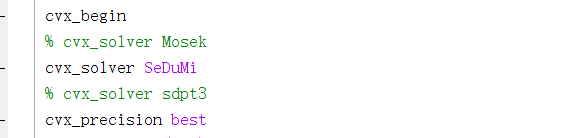# My optimization problem gradually becomes infeasible in a loopI’m using SDP and SDR in constraints, does this cause this behavior? I also found some similar posts in the forums, but it doesn’t apply.

I guess this is because computer has a limited precision. As your iteration goes on, the algorithm starts to converge, the requirements on precision become higher and higher, then something unexpected can happend.

xr,yr] = eig(AR_bar); %求矩阵的特征值和特征向量，x为特征向量矩阵，y为特征值矩阵。
eigenvaluer = diag(yr); %求对角线向量
lamdar = max(eigenvaluer); %求最大特征值
for i = 1:length(AR_bar)%求最大特征值对应的序数
if lamdar == eigenvaluer(i)
break;
end
end
y_lamdar = xr(:,i); %求矩阵最大特征值对应的特征向量
risr = sqrt(lamdar) * y_lamdar;
Risr = diag(risr);
RIsr = Risr * Risr’;
real(y_lamdar’ * RISR * y_lamdar) >= real(vr*trace(RISR));
%% T
[xt,yt] = eig(AT_bar); %求矩阵的特征值和特征向量，x为特征向量矩阵，y为特征值矩阵。
eigenvaluet = diag(yt); %求对角线向量
lamdat = max(eigenvaluet); %求最大特征值
for i = 1:length(AT_bar)%求最大特征值对应的序数
if lamdat == eigenvaluet(i)
break;
end
end
y_lamdat = xt(:,i); %求矩阵最大特征值对应的特征向量
rist = sqrt(lamdat)y_lamdat;
Rist = diag(rist);
RIst = RistRist’;
real(y_lamdat’ * RIST * y_lamdat) >= real(vt * trace(RIST));
In fact, I have a norm constraint in the program to approximate the rank 1 constraint. If this constraint is removed, the program can continue to run, but the result will be NAN and cannot converge. Is it really just about precision?

Do use Mosek. Do not use `cvx_precision best`, no matter which solver you use. if Mosek is not available to you, follow the advice at CVXQUAD: How to use CVXQUAD's Pade Approximant instead of CVX's unreliable Successive Approximation for GP mode, log, exp, entr, rel_entr, kl_div, log_det, det_rootn, exponential cone. CVXQUAD's Quantum (Matrix) Entropy & Matrix Log related functions

We have no idea what is changing on different iterations in your loop, so don’t know what is causing problems in later iterations. if you are using SCA, it is not guaranteed to converge to anything, let alone a global, or even local optimum of your original problem.